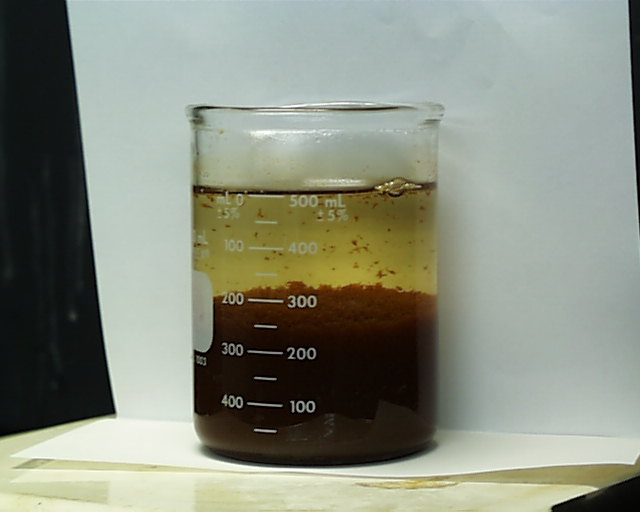# Precipitation from a Homogeneous Solution

$$\newcommand{\vecs}{\overset { \rightharpoonup} {\mathbf{#1}} }$$ $$\newcommand{\vecd}{\overset{-\!-\!\rightharpoonup}{\vphantom{a}\smash {#1}}}$$$$\newcommand{\id}{\mathrm{id}}$$ $$\newcommand{\Span}{\mathrm{span}}$$ $$\newcommand{\kernel}{\mathrm{null}\,}$$ $$\newcommand{\range}{\mathrm{range}\,}$$ $$\newcommand{\RealPart}{\mathrm{Re}}$$ $$\newcommand{\ImaginaryPart}{\mathrm{Im}}$$ $$\newcommand{\Argument}{\mathrm{Arg}}$$ $$\newcommand{\norm}{\| #1 \|}$$ $$\newcommand{\inner}{\langle #1, #2 \rangle}$$ $$\newcommand{\Span}{\mathrm{span}}$$ $$\newcommand{\id}{\mathrm{id}}$$ $$\newcommand{\Span}{\mathrm{span}}$$ $$\newcommand{\kernel}{\mathrm{null}\,}$$ $$\newcommand{\range}{\mathrm{range}\,}$$ $$\newcommand{\RealPart}{\mathrm{Re}}$$ $$\newcommand{\ImaginaryPart}{\mathrm{Im}}$$ $$\newcommand{\Argument}{\mathrm{Arg}}$$ $$\newcommand{\norm}{\| #1 \|}$$ $$\newcommand{\inner}{\langle #1, #2 \rangle}$$ $$\newcommand{\Span}{\mathrm{span}}$$$$\newcommand{\AA}{\unicode[.8,0]{x212B}}$$

If a precipitating agent is produced over a long period of time in a homogeneous solution the level of supersaturation remains low and compact crystal precipitates usually result instead of coagulated colloids. The resulting suspension of precipitate is compact, crystalline and easily filtered, whereas a precipitate formed by the addition of a precipitating agent is not easily filtered owing to a high level of relative supersaturation at the point where the reagent is added.

500 mL 0.02 M Fe2(SO4)3 was prepared and 20 mL 12 M NH4OH(aq) was added to complete the precipitation of Fe(OH)3 (s) according to the equation

$Fe^{3+} + 3 NH_4OH_{(aq)} \rightarrow Fe(OH)_3 (s) + 3 NH_4^+$The resulting solution is shown in the photograph at the left. A second sample of 500 mL 0.02 M Fe2(SO4)3 was prepared and 7.2 g urea, (NH2)2CO , was dissolved in the ferric sulfate solution. On the addition of heat the generation of ammonium hydroxide occurs according to the reaction

$(NH_2)_2CO + 3H_2O \rightarrow CO_2 + 2 NH_4OH$

After two hours at a temperature just below the boiling point, the resulting precipitate settles in a thin layer on the bottom (photo at right). This precipitate has a texture considerably different from that on the left which is flocculent and of low density. Note: this reaction seems to be pH sensitive. A first attempt at preparing the demonstration on the right included adding a small amount of hydrochloric acid to the ferric sulfate so as to assure that all ferric ion would remain in solution. After two hours of heating no precipitate had formed. From this experience one would presume that if this method were to be used as part of a quantitative analysis the pH would have to be adjusted to a point just below that which is high enough to initiate precipitation of ferric hydroxide before starting the process of heating the solution with urea added.

Here here are two time lapse videos of the process.

## Contributor

• Oliver Seely (California State University, Dominguez Hills).

This page titled Precipitation from a Homogeneous Solution is shared under a Public Domain license and was authored, remixed, and/or curated by Oliver Seely.